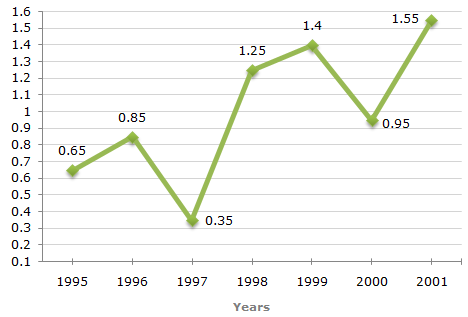# Data Interpretation - Line Charts - Discussion

### Discussion :: Line Charts - Line Chart 2 (Q.No.5)

The following line graph gives the ratio of the amounts of imports by a company to the amount of exports from that company over the period from 1995 to 2001.

Ratio of Value of Imports to Exports by a Company Over the Years.5.

In how many of the given years were the exports more than the imports ?

 [A]. 1 [B]. 2 [C]. 3 [D]. 4

Explanation:

The exports are more than the imports imply that the ratio of value of imports to exports is less than 1.

Now, this ratio is less than 1 in years 1995, 1996, 1997 and 2000.

Thus, there are four such years.

 Reddy said: (Feb 14, 2014) How you can say that ratio of value import to export is less than 1.

 Keerthireddy said: (Jun 12, 2014) Because. Here ratio=imports/exports;. They had given export value is more than import value. If smaller number divided by larger one then it will be less than 1. For eg:2/4=0.5.

 Harjeet Kaur said: (Aug 8, 2015) But we have given only the import to export ratio. So how can we determine the value of import and export?

 Vineet Gaur said: (Oct 25, 2016) This is confusing, what the ratio of exports, then only we can determine the ratio right?